## Section Exercises

1. What role does the horizontal asymptote of an exponential function play in telling us about the end behavior of the graph?

2. What is the advantage of knowing how to recognize transformations of the graph of a parent function algebraically?

3. The graph of $f\left(x\right)={3}^{x}$ is reflected about the y-axis and stretched vertically by a factor of 4. What is the equation of the new function, $g\left(x\right)$? State its y-intercept, domain, and range.

4. The graph of $f\left(x\right)={\left(\frac{1}{2}\right)}^{-x}$ is reflected about the y-axis and compressed vertically by a factor of $\frac{1}{5}$. What is the equation of the new function, $g\left(x\right)$? State its y-intercept, domain, and range.

5. The graph of $f\left(x\right)={10}^{x}$ is reflected about the x-axis and shifted upward 7 units. What is the equation of the new function, $g\left(x\right)$? State its y-intercept, domain, and range.

6. The graph of $f\left(x\right)={\left(1.68\right)}^{x}$ is shifted right 3 units, stretched vertically by a factor of 2, reflected about the x-axis, and then shifted downward 3 units. What is the equation of the new function, $g\left(x\right)$? State its y-intercept (to the nearest thousandth), domain, and range.

7. The graph of $f\left(x\right)=-\frac{1}{2}{\left(\frac{1}{4}\right)}^{x - 2}+4$ is shifted left 2 units, stretched vertically by a factor of 4, reflected about the x-axis, and then shifted downward 4 units. What is the equation of the new function, $g\left(x\right)$? State its y-intercept, domain, and range.

For the following exercises, graph the function and its reflection about the y-axis on the same axes, and give the y-intercept.

8. $f\left(x\right)=3{\left(\frac{1}{2}\right)}^{x}$

9. $g\left(x\right)=-2{\left(0.25\right)}^{x}$

10. $h\left(x\right)=6{\left(1.75\right)}^{-x}$

For the following exercises, graph each set of functions on the same axes.

11. $f\left(x\right)=3{\left(\frac{1}{4}\right)}^{x}$, $g\left(x\right)=3{\left(2\right)}^{x}$, and $h\left(x\right)=3{\left(4\right)}^{x}$

12. $f\left(x\right)=\frac{1}{4}{\left(3\right)}^{x}$, $g\left(x\right)=2{\left(3\right)}^{x}$, and $h\left(x\right)=4{\left(3\right)}^{x}$

For the following exercises, match each function with one of the graphs pictured below.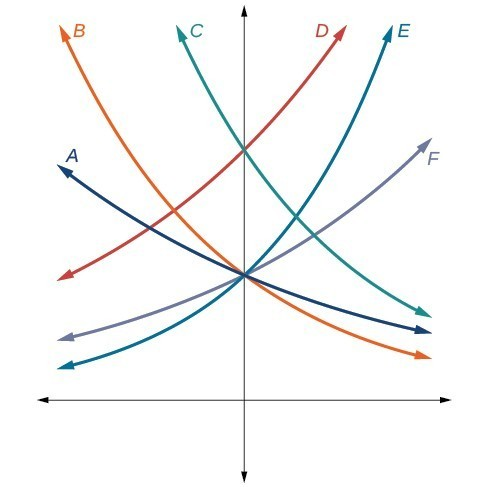13. $f\left(x\right)=2{\left(0.69\right)}^{x}$

14. $f\left(x\right)=2{\left(1.28\right)}^{x}$

15. $f\left(x\right)=2{\left(0.81\right)}^{x}$

16. $f\left(x\right)=4{\left(1.28\right)}^{x}$

17. $f\left(x\right)=2{\left(1.59\right)}^{x}$

18. $f\left(x\right)=4{\left(0.69\right)}^{x}$

For the following exercises, use the graphs shown below. All have the form $f\left(x\right)=a{b}^{x}$.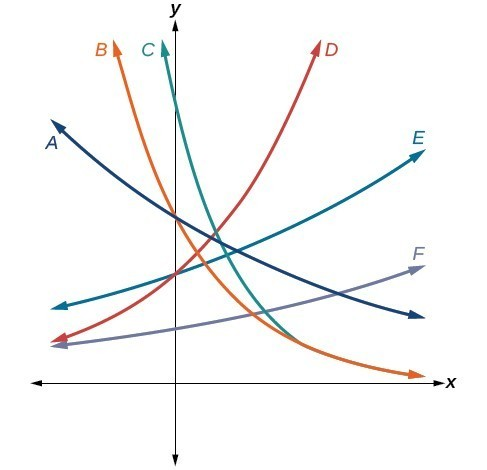19. Which graph has the largest value for b?

20. Which graph has the smallest value for b?

21. Which graph has the largest value for a?

22. Which graph has the smallest value for a?

For the following exercises, graph the function and its reflection about the x-axis on the same axes.

23. $f\left(x\right)=\frac{1}{2}{\left(4\right)}^{x}$

24. $f\left(x\right)=3{\left(0.75\right)}^{x}-1$

25. $f\left(x\right)=-4{\left(2\right)}^{x}+2$

For the following exercises, graph the transformation of $f\left(x\right)={2}^{x}$. Give the horizontal asymptote, the domain, and the range.

26. $f\left(x\right)={2}^{-x}$

27. $h\left(x\right)={2}^{x}+3$

28. $f\left(x\right)={2}^{x - 2}$

For the following exercises, describe the end behavior of the graphs of the functions.

29. $f\left(x\right)=-5{\left(4\right)}^{x}-1$

30. $f\left(x\right)=3{\left(\frac{1}{2}\right)}^{x}-2$

31. $f\left(x\right)=3{\left(4\right)}^{-x}+2$

For the following exercises, start with the graph of $f\left(x\right)={4}^{x}$. Then write a function that results from the given transformation.

32. Shift f(x) 4 units upward

33. Shift f(x) 3 units downward

34. Shift f(x) 2 units left

35. Shift f(x) 5 units right

36. Reflect f(x) about the x-axis

37. Reflect f(x) about the y-axis

For the following exercises, each graph is a transformation of $y={2}^{x}$. Write an equation describing the transformation.

38.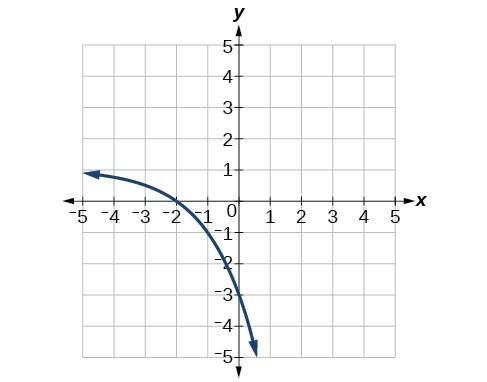39.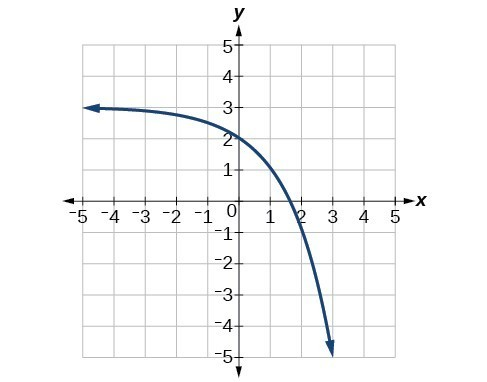40.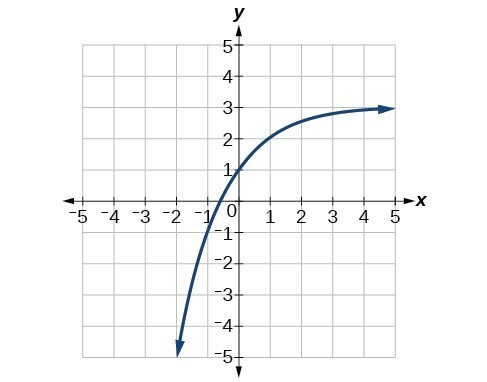For the following exercises, find an exponential equation for the graph.

41.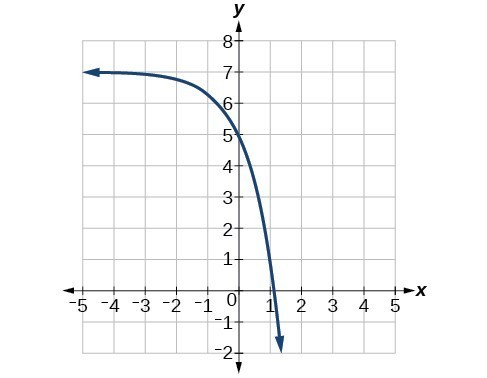42.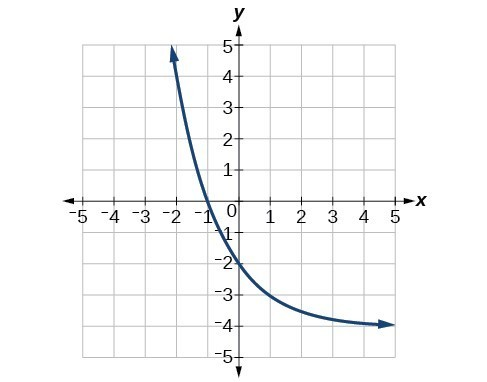For the following exercises, evaluate the exponential functions for the indicated value of x.

43. $g\left(x\right)=\frac{1}{3}{\left(7\right)}^{x - 2}$ for $g\left(6\right)$.

44. $f\left(x\right)=4{\left(2\right)}^{x - 1}-2$ for $f\left(5\right)$.

45. $h\left(x\right)=-\frac{1}{2}{\left(\frac{1}{2}\right)}^{x}+6$ for $h\left(-7\right)$.

For the following exercises, use a graphing calculator to approximate the solutions of the equation. Round to the nearest thousandth. $f\left(x\right)=a{b}^{x}+d$.

46. $-50=-{\left(\frac{1}{2}\right)}^{-x}$

47. $116=\frac{1}{4}{\left(\frac{1}{8}\right)}^{x}$

48. $12=2{\left(3\right)}^{x}+1$

49. $5=3{\left(\frac{1}{2}\right)}^{x - 1}-2$

50. $-30=-4{\left(2\right)}^{x+2}+2$

51. Explore and discuss the graphs of $F\left(x\right)={\left(b\right)}^{x}$ and $G\left(x\right)={\left(\frac{1}{b}\right)}^{x}$. Then make a conjecture about the relationship between the graphs of the functions ${b}^{x}$ and ${\left(\frac{1}{b}\right)}^{x}$ for any real number $b>0$.

52. Prove the conjecture made in the previous exercise.

53. Explore and discuss the graphs of $f\left(x\right)={4}^{x}$, $g\left(x\right)={4}^{x - 2}$, and $h\left(x\right)=\left(\frac{1}{16}\right){4}^{x}$. Then make a conjecture about the relationship between the graphs of the functions ${b}^{x}$ and $\left(\frac{1}{{b}^{n}}\right){b}^{x}$ for any real number n and real number $b>0$.

54. Prove the conjecture made in the previous exercise.